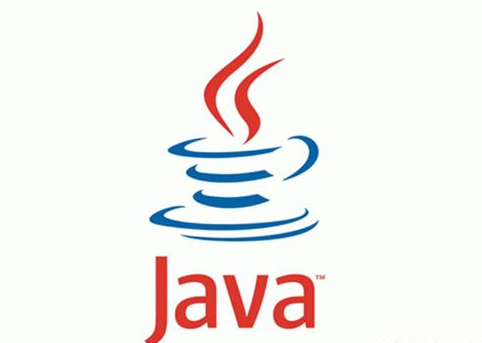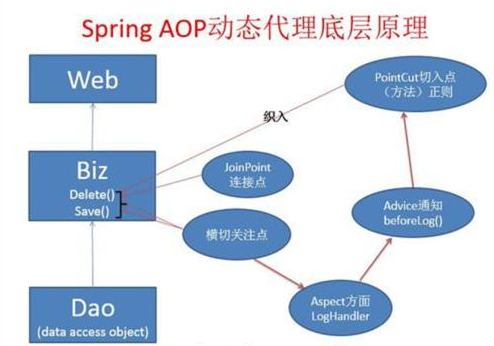long begin = System.currentTimeMillis();

try {

// .... 具体的代码段

} finally {

long end= System.currentTimeMillis();

System.out.println("cost: " + (end - begin));

}@Pointcut("execution(public * namespace.*.*(*))") public void point() { } @Around("point()") public Object doAround(ProceedingJoinPoint joinPoint) throws Throwable { long start = System.currentTimeMillis(); try{ return joinPoint.proceed(); } finally {

long end= System.currentTimeMillis();

System.out.println("cost: " + (end - begin));

} }

AOP的机制入下图：